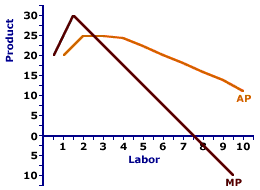Saturday  January 28, 2023
 AmosWEB means Economics with a Touch of Whimsy!LONG-RUN AGGREGATE MARKET: A macroeconomic model relating the price level and real production under the assumption that ALL prices flexible. This is one of two aggregate market submodels used to analyze business cycles, aggregate production, unemployment, inflation, stabilization policies, and related macroeconomic phenomena. The other is the short-run aggregate market. The long-run aggregate market isolates the interaction between aggregate demand and long-run aggregate supply. The key assumption of this model is that ALL prices, especially resource prices, are flexible. The primary result of this model is that the economy achieves long-run equilibrium at full-employment real production.AVERAGE-MARGINAL RELATION:

A mathematical connection between a marginal value and the corresponding average value stating that the change in the average value depends on a comparison between the average and the marginal. This mathematical relation between average and marginal surfaces throughout the study of economics, especially production (average product and marginal product), cost (average total cost and marginal cost), and revenue (average revenue and marginal revenue). A similar relation is that between a total value and the corresponding marginal value.
The mathematical relation between average and marginal means that the average value is "driven" by the marginal value.
• If the marginal is less than the average, then the average declines.

• If the marginal is greater than the average, then the average rises.

• If the marginal is equal to the average, then the average does not change.
The reason for this relation is that average value is based on the existing situation that is then modified by the marginal value. This average-marginal relation applies to average and marginal product, average and marginal cost, average and marginal revenue, and well, any other average and marginal encountered in the study of economics.

To illustrate the basic nature of the average-marginal relation consider an example. Suppose that there is a room containing five people that have been painstakingly and accurately measured for height. The average height of this group is 66 inches (5' 6"). Some are taller than 5' 6" and some are shorter, but the average is 5' 6".

What happens to this 5' 6" average should a sixth person enter the room? This surely depends on the height of this extra person, this marginal addition to this group, does it not?

• If this "marginal" person is 6' tall, then the group's average rises to exactly 5' 7". The marginal is greater than the average, and the average rises.

• If the marginal sixth person is, however, a mere 5' tall, the marginal is less than the average, then the average declines to 5' 5".

• And if the new, marginal person, is exactly 5' 6'', the same as the existing average, then the average does not change.
Average and Marginal ProductThis average-marginal relation can be graphically illustrated, using the average product and marginal product curves displayed to the right. A comparison between average product and marginal product reveals three alternatives.
• When the marginal measure is greater than the average measure (that is, the marginal product curve lies above the average product curve), then the average measure increases (and the average product curve has a positive slope).

• Alternatively, when the marginal measure is less than the average measure (that is, the marginal product curve lies below the average product curve), then the average measure decreases (and the average product curve has a negative slope).

• In addition, when the marginal measure is equal to the average measure (that is, the marginal product curve intersects the average product curve), then the average measure does not change (and the average product curve has a zero slope).

 <= AVERAGE FIXED COST CURVE AVERAGE PHYSICAL PRODUCT =>Recommended Citation:

AVERAGE-MARGINAL RELATION, AmosWEB Encyclonomic WEB*pedia, http://www.AmosWEB.com, AmosWEB LLC, 2000-2023. [Accessed: January 28, 2023].

Check Out These Related Terms...

Or For A Little Background...

And For Further Study...
Search Again?BROWN PRAGMATOX[What's This?] Today, you are likely to spend a great deal of time flipping through the yellow pages looking to buy either a genuine down-filled comforter or a 200-foot blue garden hose. Be on the lookout for a thesaurus filled with typos.Your Complete ScopeThe wealthy industrialist, Andrew Carnegie, was once removed from a London tram because he lacked the money needed for the fare."Even a mistake may turn out to be the one thing necessary to a worthwhile achievement."-- Henry FordBCUABusiness Computers Users AssociationA PEDestrian's Guide Xtra CreditTell us what you think about AmosWEB. Like what you see? Have suggestions for improvements? Let us know. Click the User Feedback link.| | | | | | | | | | |
| | | |

Thanks for visiting AmosWEB